Reach Us+44-7482-875032
Mathematica and LaTeX Integration | OMICS International
Journal of Applied & Computational Mathematics
All submissions of the EM system will be redirected to Online Manuscript Submission System. Authors are requested to submit articles directly to Online Manuscript Submission System of respective journal.

# Mathematica and LaTeX Integration

Andreoli M*

Department of Physics, University of Pisa and INFN-Pisa Section, Italy

Corresponding Author:
Andreoli M
Department of Physics
University of Pisa and INFN-Pisa Section
Largo Pontecorvo 3, I-56127 Pisa, Italy
Tel: +390502214000
E-mail: [email protected]

Received Date: May 28, 2016; Accepted Date: June 16, 2016; Published Date: June 22, 2016

Citation: Andreoli M (2016) Mathematica and LaTeX Integration. J Appl Computat Math 5:308. doi:10.4172/2168-9679.1000308

Copyright: © 2016 Andreoli M. This is an open-access article distributed under the terms of the Creative Commons Attribution License, which permits unrestricted use, distribution, and reproduction in any medium, provided the original author and source are credited.

Visit for more related articles at Journal of Applied & Computational Mathematics

#### Abstract

This document is about Mathex, a software package that allows to embed Mathematica © macro expressions into a LaTeX document, combining together the power of the two systems. It works in this way: when You click F2 on your preferred editor, before of the TeX to PDF conversion, a pre-processor (written in the PERL language) call Mathematica, do the computation globally (i.e. treating the file as a single program) and replaces the macros in the final TeX. The macros should be inserted between the two conventional simbols <% ... %>, and can contain every valid Mathematica’s expression, or more espressions, separated by semicolon. As every expression and every graphics object get created everytime on-the-fly, the process can be slow.

#### Keywords

Mathex; Mathematica; Symbol; Algebraic expression.

#### Expressions

The Mathematica expressions should be inserted in the form of a macro, i.e with blocks like:

<% expr; expr;...; expr | {opt->value, opt->value,...} %>

Blocks can span over many rows, creating complex expressions or even complete Mathematica modules.

A macro, usually, creates some mathematical object but it can also output a final result.

If the last espression is followed by a semicolon, as usual for Mathematica, no output will be produced.

Example: the following macro :

<%Clear[f,g,h]; w=5; f[x_]:=1/(x^2-Sin[w*x]); f[x] %>

1. Initialize the variables f,g,h.

2. Create the global variable w.

3. Define the function f(x).

4. Embed the last result (in this case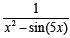) in to the TeX final code.

You can use the greek symbols in the standard Mathematica syntax. For example:

<%\\[Omega]^3 /(\\[Nu]+\\[Mu])%>

will produce: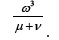#### Special Formattings

A macro <% ... %> can have a (limited) number of options (opt- >value) after the ’|’ symbol. An option modify some secondary aspects of output:

Examples:

1. Characters dimension: <% x^3/(1-x) | tiny %>: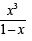2. Formatting: <% Sin[x]| bold%>: sin(x) ,

3. Box <% 1/Pi | box %>: 1/π

The most important is the scale option, that allow to change the size (Figure 1):

<% Plot[ Sin[x]/x,{x,-2Pi,2Pi}] | scale->0.4 %>:

#### Non-standard Evaluation

You can ask Mathematica to left unevaluated an expression, adding the keyword //HoldForm:

G= <% Sum[ x^n, {n,0,Infinity}] //HoldForm %>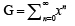This allow to use the Mathematica engine in order to compose complex formulas, that otherwise would be reduced and/or simplified.

#### Some Algebra

Starting with the previously-defined functions: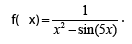and with the Taylor’s formula of order 21: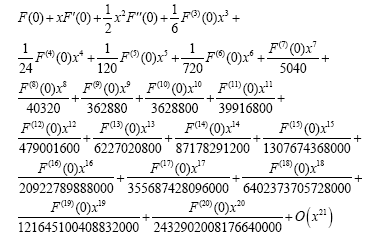we have: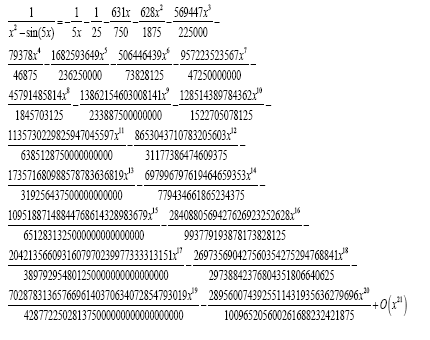The serie expansion of its 6th power is: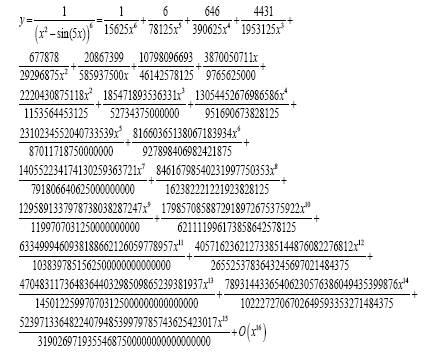Its 5th derivative, that we will insert in this way:

<% D[ f[x],{x,5}] %>:

is: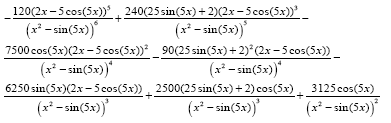and its definite integral between 1 and 2π is: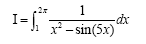that, evaluated numerically, give us I=0.874779.

Now, we generate the following array: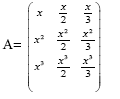and will apply f(x) on it, obtaining the new array: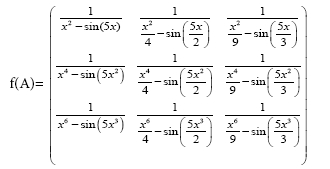We can compute the value of its determinants in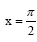, obtaining :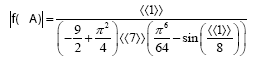Algebraic expressions with large output are not a problem, neither for Mathematica, nor for TeX :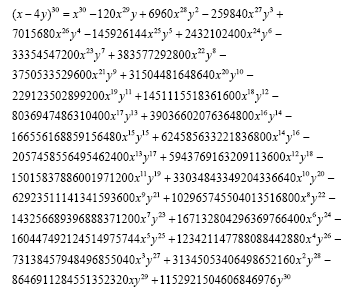We consider, now, the following integral function: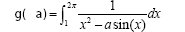and expand it in Taylor’s serie: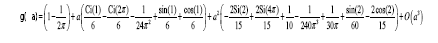, or, in numerical form:

g( a) = 0.840845 + 0.286068(a + 0.) + 0.166017(a + 0.)2 +O((a + 0.)3 ) ,

Obviously, you can access the enourmous collection of functions of Mathematica. So, if You need the first 20 Chebyshev’s polynomials Tn(x), You just can ask:

<% Table[ ChebyshevT[n,x],{n,1,20}] | small %>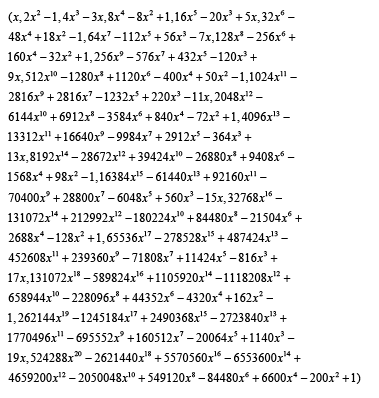#### Graphics

The system creates a graphic file if the last expression of the macro produces a Mathematica Graphics[] object. You can obtain the simplest graphs directly from the standard plotting functions (Figures 2 and 3):

<% opt={scale->0.5}; Plot[ {x,x^2,x^3},{x,-2Pi,2Pi}] %>

but You can have more elaborated graphs merging Mathematica graphic primitives (Figures 4-6):

<%opt={scale->0.4}; GraphPlot[Table[i -> Mod[i^2, 102], {i, 0, 102}]] %>

or 3D graph:

or histograms:

etc.

Each graphs file is saved in the directory ./eps, under the current path.

For the Mathematica graphical primitives, please consults a Mathematica book.

#### Caching

With the efficiency in mind, the system call Mathematica only if needed. For example, if You change only the text and no equations at all, the program will try to reuse the previous results. This caching activity is a sperimental feature, so it is possible to disable it, adding the following directive:

<% NOCACHE=1; %>

#### Requirements

This sofware is available (and tested) only on the Linux Operating System, and its derivatives.

It requires [3,4]:

1. Wolfram’s Mathematica installed (tested versions form 8 to 10)

2. Latex typesetting system

3. Perl (version >5) with modules: JSON; Digest::MD5.

4. Lyx, Emacs, or Vim (for editing)

5. A pdf viewer (evince, atril, okular, qpdfview, acroread, gv, xpdf, ....)

6. kdialog and xedit (strongly suggested)

### Installation

Please, download the latest version of this package from the following url:

http://github.com/michele.andreoli/mathex/

Once downloaded the tgz package in a temporary directory, you can run these commands:

# tar -zxf mathex-VERSION.tgz

#./install.sh

The install.sh command copy the files in the installation directory (usually \$HOME/.math) and integrate that software with the supported editors (Lyx, Emacs and Vim).

#### Customization

Mathex calls Mathematica via a script wrapper. You can find the script (called math.run) in the installation directory. A little tuning allows to use local (or sudo) installation, or also remote Mathematica host (via ssh).

#### Usage

If You are using a supported editor, the <F2> shortcut should be available. As alternative, you can pre-process by yourself with the command:

#/path/to/replace.pl file.in > file.out

As the Lyx editor is concerned, we suggest to insert the macros <% ...%> using the Lyx TeX blocks (with Control-L) (Figure 7).

#### Disclaimer

The Mathex package (the PERL code and BASH scripts contained) is copyrighted (©)2004-2016 Michele Andreoli. It is distributed under the GNU Free Documentation License.

Of course, I disavow any potential liability for the contents of this document.

Use of the concepts, examples, and/or other content of this document and related software is entirely at your own risk.

#### References

Select your language of interest to view the total content in your interested language

### Article Usage

• Total views: 10635
• [From(publication date):
June-2016 - Aug 20, 2019]
• Breakdown by view type
• HTML page views : 10466
• PDF downloads : 169

## Post your commentCan't read the image? click here to refresh
###### Peer Reviewed Journals

Make the best use of Scientific Research and information from our 700 + peer reviewed, Open Access Journals

International Conferences 2019-20

Meet Inspiring Speakers and Experts at our 3000+ Global Annual Meetings

Top